# A.2 Rigid transforms

Rigid transforms are used to specify both the transformation of points and vectors between coordinate frames, as well as the relative position and orientation between coordinate frames.Figure A.3: A position vector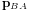and rotation matrix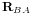describing the position and orientation of frame B with respect to frame A.

Consider two 3D coordinate frames in space, A and B (Figure A.3). The translational position of B with respect to A can be described by a vectorfrom the origin of A to the origin of B (described with respect to frame A). Meanwhile, the orientation of B with respect to A can be described by the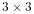rotation matrix(Section A.1). The combined position and orientation of B with respect to A is known as the pose of B with respect to A.Figure A.4: Point vectors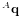and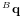describing the position of a point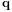with respect to frames A and B.

Now, assume we have a 3D point, and consider its coordinates with respect to both frames A and B (Figure A.4). Given the pose descriptions given above, it is fairly straightforward to show that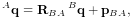(A.10)

and, given (A.2), that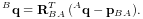(A.11)

If we extend our points into a 4D homogeneous coordinate space with the fourth coordinate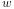equal to 1, i.e.,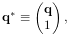(A.12)

then (A.10) and (A.11) can be simplified to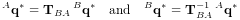where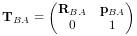(A.13)

and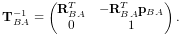(A.14)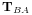is the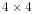rigid transform matrix that transforms points from B to A and also describes the pose of B with respect to A (Figure A.5).Figure A.5: The transform matrixfrom B to A.

It is straightforward to show that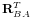and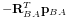describe the orientation and position of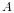with respect to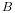, and so therefore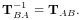(A.15)

Note that if we are transforming a vector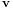instead of a point between B and A, then we are only concerned about relative orientation and the vector transforms (A.3) and (A.4) should be used instead. However, we can express these usingif we embed vectors in a homogeneous coordinate space with the fourth coordinateequal to 0, i.e.,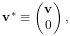(A.16)

so that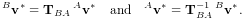Figure A.6: Three coordinate frames A, B, and C and the transforms relating each one to the other.

Finally, we consider transform composition. Suppose we have three coordinate frames, A, B, and C, each related to the other by transforms,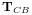, and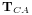(Figure A.6). Using the same reasoning used to derive (A.6) and (A.9), it is easy to show that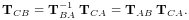(A.17)